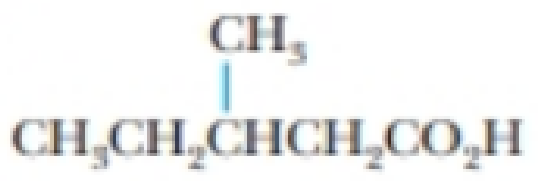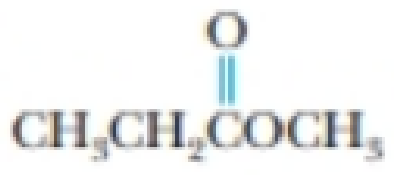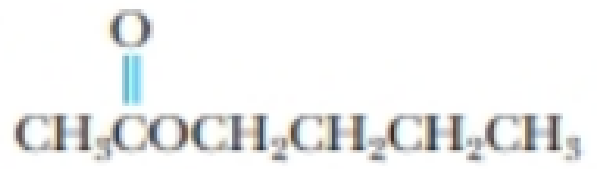Chapter 23, Problem 57PS

Chapter
Section
Textbook Problem

Identify the class of each of the following compounds, and give the systematic name for each:(a)(b)(c)(d)(a)

Interpretation Introduction

Interpretation: The name of the given compund should be given and the class of the compund has to be identified.

Concept introduction:

Carboxylic acid: One OH group is attached to the carbonyl carbon atom of the compound. It is represented as RCOOH

The IUPAC name for the carboxylic acid derivatives is written by replcing the ‘e’ of alkanes from “–oic acid”.

Explanation

The given compound is,

The parent name will be pentane because the maximum number of carbon atom in longest carbon chain are five...

(b)

Interpretation Introduction

Interpretation: The name of the given compund should be given and the class of the compund has to be identified.

Concept introduction:

Ester: One OR' group is attached to the carbonyl carbon atom of the compound. It is represented as RCOOR'. The hydrogen atom of COOH is replaced by an alkyl group.

The IUPAC name for the esters is written by replcing the ‘e’ of alkanes from ‘oate’.

(c)

Interpretation Introduction

Interpretation: The name of the given compund should be given and the class of the compund has to be identified

Concept introduction:

Ester: One OR' group is attached to the carbonyl carbon atom of the compound. It is represented as RCOOR'. The hydrogen atom of COOH is replaced by an alkyl group.

The IUPAC name for the esters is written by replcing the ‘e’ of alkanes from ‘oate’.

(d)

Interpretation Introduction

Interpretation: The name of the given compund should be given and the class of the compund has to be identified

Concept introduction:

Carboxylic acid: One OH group is attached to the carbonyl carbon atom of the compound. It is represented as RCOOH

The IUPAC name for the carboxylic acid derivatives is written by replcing the ‘e’ of alkanes from “–oic acid”.

Still sussing out bartleby?

Check out a sample textbook solution.

See a sample solution

The Solution to Your Study Problems

Bartleby provides explanations to thousands of textbook problems written by our experts, many with advanced degrees!

Get Started

Find more solutions based on key concepts